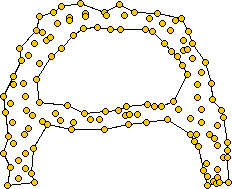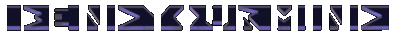# Everything You Always Wanted to Know About Alpha Shapes But Were Afraid to Ask## What are alpha shapes?

The concept of alpha shapes is an approach to formalize the intuitive notion of "shape" for spatial point sets. The Alpha shape is a concrete geometric concept which is mathematically well defined: it is a generalization of the convex hull and a subgraph of the Delaunay triangulation. Given a finite point set, a family of shapes can be derived from the Delaunay triangulation of the point set; a real parameter, "alpha," controls the desired level of detail. The set of all real alpha values leads to a whole family of shapes capturing the intuitive notion of "crude" versus "fine" shapes of a point set.

Here is an example for different values of alpha. Notice the second picture which displays the boundary of the point set's convex hull.## Formal definition

First, let us define a generalized disk of radius 1/alpha to be the following:

• If alpha > 0, it is an ordinary closed disk of radius 1/alpha
• If alpha = 0, it is a halfplane
• If alpha < 0, it is the complement of a closed disk of radius -1/alpha

Then, given a set of points and a specific value for alpha, we construct the alpha shape graph in the following way:

1. For each point Pi in our point set, we create a vertex Vi.
2. We create an edge between two vertices Vi and Vj whenever there exists a generalized disk of radius 1/alpha containing the entire point set and which has the property that Pi and Pj lie on its boundary.

## Applet Instructions

• Insert & drag points using the left mouse button
• Delete a point using the right mouse button
• Try different values for alpha by gliding the bottom cursor
• The top-left corner displays the generalized disk which corresponds to the current value for alpha
• Turn on or off the various checkboxes in order to display other informations
• Try out the demo!! (you may have to wait a few seconds before it starts due to file downloading).